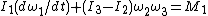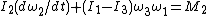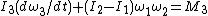# Euler's equations of motion

## Euler's equations of motion

A set of three differential equations expressing relations between the force moments, angular velocities, and angular accelerations of a rotating rigid body. They are equations of motion in the usual dynamical sense, of forms (1)–(3).

(1)(2)(3)The formulation employs as coordinate axes the three principal axes of rotational inertia of the body that can rotate about a body-fixed point, which is the center of mass if constraints are absent. These reference axes, which form a righthand set, are indicated by subscripts 1, 2, and 3 in the equations, where I1, I2, and I3 represent the principal moments of inertia; ω1, ω2, and ω3 the angular velocities about the axes; M1, M2, and M3 the corresponding force moments; and t the time. See Rigid-body dynamics

McGraw-Hill Concise Encyclopedia of Physics. © 2002 by The McGraw-Hill Companies, Inc.
Mentioned in ?
References in periodicals archive ?
developed the analytic solutions for a TSS subjected to internal tether tension moment and external aerodynamic torque for spin-up and spin-down maneuvers by the approximation of Euler's equations of motion via Fresnel integrals and sine and cosine integrals .

Site: Follow: Share:
Open / Close